Introduction

Basics of ggmosaic

• designed to create visualizations of categorical data
• can produce bar charts, stacked bar charts, mosaic plots, and double decker plots
• plots are constructed hierarchically, so the ordering of the variables is very important.
• integrated in ggplot2 as a geom which allows for facetting and layering

Creation of ggmosaic

ggmosaic was created primarily using ggproto and the productplots package

ggproto allows you to extend ggplot2 from within your own packages

• ggmosaic began as a geom extension of the rect geom
• used the data handling provided in the productplots package
• calculates xmin, xmax, ymin, and ymax for the rect geom to plot

ggplot2 limitations

ggplot2 is not capable of handling a variable number of variables

• current solution: read in the variables x1 and x2 as x = product(x1, x2)

• product function:
• a wrapper function for a list
• allows for it to pass check_aesthetics

These limitations also lead to issues with the labeling, but those can be fixed manually.

geom_mosaic: setting the aesthetics

Aesthetics that can be set:

• weight : select a weighting variable
• x : select variables to add to formula
• declared as x = product(x1, x2, …)
• fill : select a variable to be filled
• if the variable is not also called in x, it will be added to the formula in the first position
• conds : select a variable to condition on
• declared as conds = product(cond1, cond2, …)

These values are then sent through productplots functions to create the formula for the desired distribution

Formula: weight ~ fill + x | conds

From the aesthetics to the formula

Example of how the formula is built

• weight = 1
• x = product(Y, X)
• fill = W
• conds = product(Z)

These aesthetics set up the formula for the distribution:

Formula: 1 ~ W + X + Y | Z

Because a mosaic plot is constructed hierarchically through alternating spines, the ordering of the variables is very important.

1 ~ X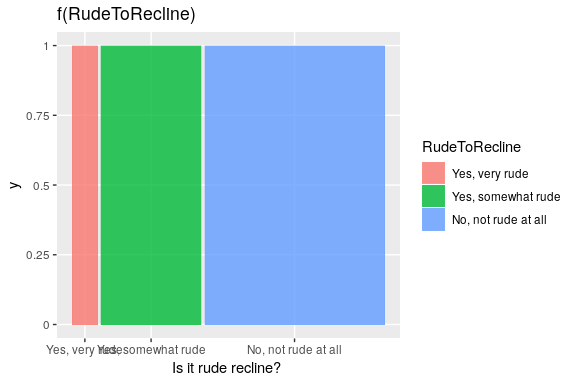1 ~ Y + X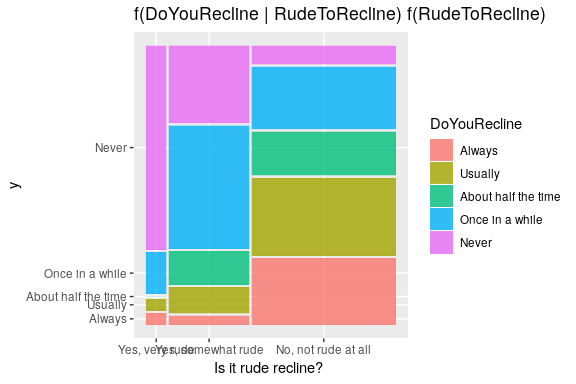1 ~ X + Y / Z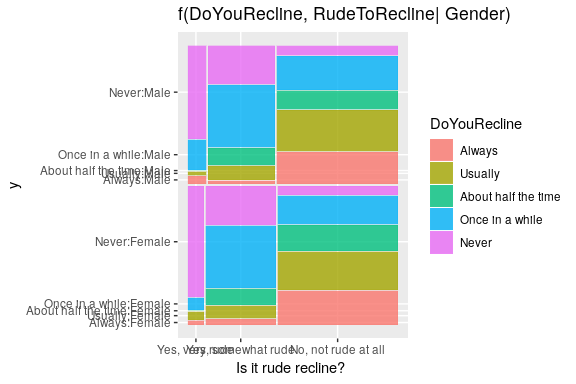Alternative to conditioning: facetting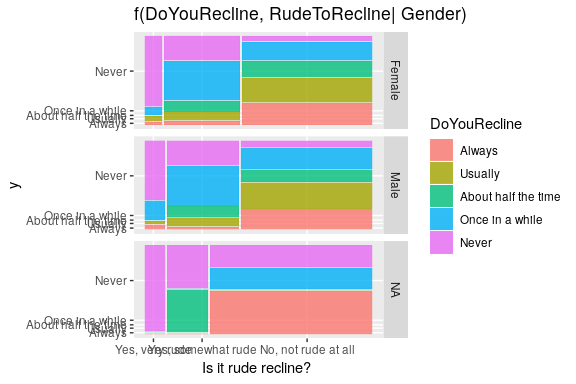Importance of ordering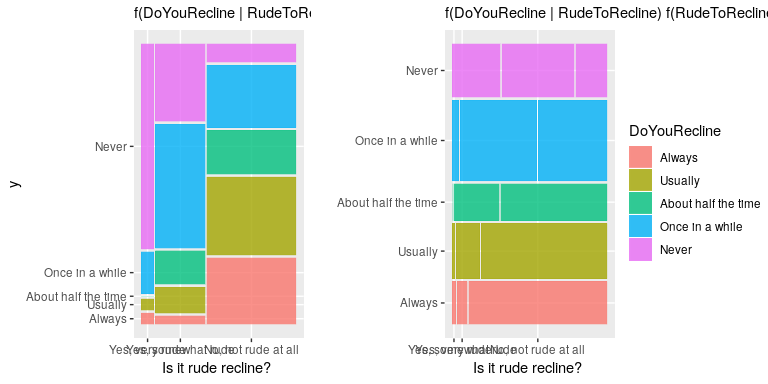Other features of geom_mosaic

Arguments unique to geom_mosaic:

• divider: used to declare the type of partitions to be used
• offset: sets the space between the first spine

Divider function: Types of partitioning

Four options available for each partion:

• vspine: width constant, height varies.
• hspine: height constant, width varies.
• vbar: height constant, width varies.
• hbar: width constant, height varies.

geom_mosaic: offset

offset: Set the space between the first spine

• default = 0.01
• space between partitions decreases as layers build